# Principal Governing Equations

 |   Tiled Menu   |   Tables of Content   |  Banner Video   |  Tohline Home Page   |

According to the eloquent discussion of the broad subject of Fluid Mechanics presented by Landau and Lifshitz (1975), the state of a moving fluid is determined by five quantities: the three components of the velocity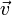$~\vec{v}$ and, for example, the pressure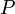$~P$ and the density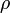$~\rho$ . For our discussions of astrophysical fluid systems throughout this Hypertext Book [H_Book], we will add to this the gravitational potential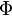$~\Phi$. Accordingly, a complete system of equations of fluid dynamics should be six in number. For an ideal fluid these are:

Equation of Continuity
(Mass Conservation)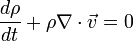$\frac{d\rho}{dt} + \rho \nabla \cdot \vec{v} = 0$

Euler Equation
(Momentum Conservation)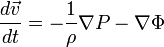$\frac{d\vec{v}}{dt} = - \frac{1}{\rho} \nabla P - \nabla \Phi$

First Law of Thermodynamics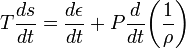$T \frac{ds}{dt} = \frac{d\epsilon}{dt} + P \frac{d}{dt} \biggl(\frac{1}{\rho}\biggr)$

Poisson Equation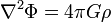$\nabla^2 \Phi = 4\pi G \rho$

In the 1st Law of Thermodynamics,$~T$ is the temperature,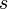$~s$ is the specific entropy,$~\epsilon$ is the specific internal energy of the gas; and in the Poisson equation,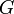$~G$ is the Newtonian gravitational constant. These differential equations relate the spatial and temporal variations of the principal variables to one another in a physically consistent fashion. Temporal variations are accounted for through first-order derivatives with respect to time,$~t$, and variations in space,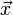$~\vec{x}$, are accounted for through the differential operators: the gradient, divergence, and Laplacian.

By restricting our discussions to physical systems that are governed by this set of equations, for the most part we will be considering the structure, stability, and dynamical behavior of compressible, inviscid fluid systems that are self-gravitating. We will assume that no electromagnetic forces act on the fluid (e.g., the effects of magnetic fields on an ionized plasma fluid will not be considered). Also, in the absence of dynamically generated shocks, we will usually assume that all compressions and rarefactions occur adiabatically (i.e.,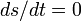$~ds/dt = 0$), in which case the third governing equation identified above will be replaced by the

Adiabatic Form of the
First Law of Thermodynamics

(Specific Entropy Conservation)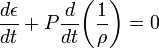$~\frac{d\epsilon}{dt} + P \frac{d}{dt} \biggl(\frac{1}{\rho}\biggr) = 0$ .

[BLRY07], p. 14, Eq. (1.60)

To complete the description of any specific astrophysical system, this set of differential equations must be supplemented by additional relations — for example, a relationship between$~\epsilon$ and the other two state variables,$~P$ &$~\rho$ — which (at least in the context of this H_Book) usually will be algebraic expressions motivated by the specific physics that is relevant to the chosen system.

## Related Wikipedia Discussions

 © 2014 - 2019 by Joel E. Tohline |   H_Book Home   |   YouTube   | Context: | PGE | SR | Appendices: | Equations | Variables | References | Binary Polytropes | Ramblings | Images | Images (2016 Layout) | ADS |